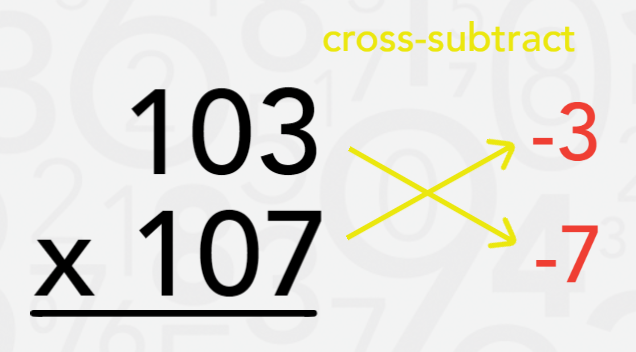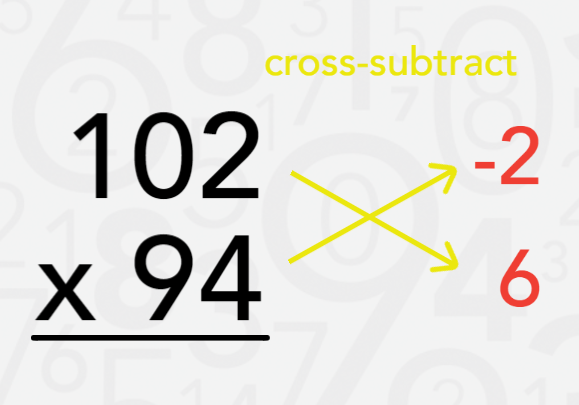# Mathnasium #MathTricks: Number Sense (Multiplication Part 3)

By Mathnasium | Added Feb 22, 2023Welcome to Mathnasium’s Math Tricks series. Today we are multiplying numbers that are near one hundred.

We can use mental math to quickly multiply numbers that are slightly smaller or larger than one hundred using three simple steps:

Step 1: Subtract each of the numbers from 100.
Step 2: “Cross-subtract” and write the answer.
Step 3: Multiply the two differences from Step 1 and write the answer beside the number from Step 2. (If the product is negative, add it to one hundred times the number from Step 2.)

Follow the examples below to find each product.

##### Example 1: Find 103 x 107.

Step 1: Subtract each of the numbers from 100.
100 minus 103 is -3.
100 minus 107 is -7.

##### Step 2: “Cross-subtract” the first number and the second difference OR the second number and the first difference from Step 1.

103 minus the second difference of -7 is 110.
Alternatively, 107 minus the first difference of -3 is also 110.##### Step 3: Multiply the two differences from Step 1.

The product of the two differences is -3 x -7 = 21.

##### Step 4: Write the final answer.

The first part of the final answer is the difference from Step 2: 11000.
The last part of the final answer is the product from Step 3: 11021.

##### Step 1: Subtract each of the numbers from 100.

100 minus 102 is -2.
100 minus 94 is 6.

##### Step 2: “Cross-subtract” the first number and the second difference OR the second number and the first difference from Step 1.

102 minus the second difference of 6 is 96.
Alternatively, 94 minus the first difference of -2 is also 96.##### Step 3: Multiply the two differences from Step 1.

The product of the two differences is 6 x -2 = -12.

##### Step 4: Write the final answer.

The first part of the final answer is the difference from Step 2: 9600.
The last part of the final answer is the product from Step 3. Since this product is negative, we add it to one hundred times the number from Step 2:

9600 + (-12) = 9,588.